Applets for Calculus

Visualizing Functions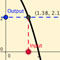Visualizing Functions as Graphs 1.1 A graph of a functions is a visual representation of the pairs (input, output), in the plane. This applet will help you to understand the connection between the graph of a function and a function as and input-output machine.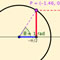Graphs of Sine and Cosine 1.2 An applet illustrating how the graphs of sine and cosine are related to the unit circle.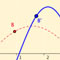Transformations of Functions 1.3 An applet illustrating how transformations affect the graph of a function. Transformations are represented both algebraically and graphically.

Differential Calculus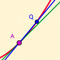Construction of the Tangent to a Parabola 2.1 An applet illustrating how the tangent to a parabola is obtained through successive secant lines.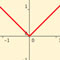Functions with Corners 2.1 Some examples of graphs with corners which I talked about in lecture. The tangent line to a graph does not exist at a corner.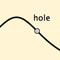Algebraic Simplification 2.3 When computing the limit of a function at a point it is sometimes necessary to do some algebraic simplification of the function before you can substitute in the value. What we are actually doing is replacing the function with another function which is equal to it but has a larger domain (i.e. the simplified function has the value x=a in its domain). We can think of this as filling in the hole on the original function.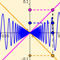Squeeze Theorem Examples 2.3 The Squeeze Theorem is a very useful theorem which allows you to compute limits of some trickier functions. This applet allows you to explore the Squeeze Theorem visually. Here is another example.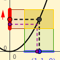Epsilon-Delta Definition of Limit 2.4 The epsilon-delta definition of the limit can be difficult to grasp at first. This is an interactive illustration of the epsilon-delta game to help get your head around what is going on.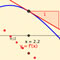The Derivative of Sine 3.3 So you know the derivative of sine is cosine, but do you know why? This applet gives some pretty compelling visual evidence to suggest why. Of course, a rigorous argument would rely on applying the definition of derivative.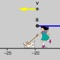Motion of a Particle in 1-Dimension 3.7 If a particle has negative acceleration then it must be slowing down, right? Nope. Sorry. Try again. This is an extremely common misconception. This applet is intended to address this misconception and help you understand what the sign of the first and second derivative is telling you. The sign of the first derivative indicates the direction of motion, whereas the sign of the second derivatice indicates the direction of the acceleration. A partical is slowing down if it is accelerating in the opposite direction from which it is moving. This means we also have to know its direction of motion to conclude anything about whether it is speeding up or slowing down.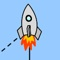Related Rates - Tracking a Rocket 3.9 A visualization of a classic related rates problem - a radar station tracking a launching rocket.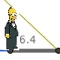Related Rates - Man's Shadow 3.9 A visualization of a classic related rates problem - a man walking away from a lamppost.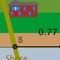Related Rates - Lighthouse Beam 3.9 A visualization of a classic related rates problem - a beam of light from a lighthouse is traveling along the shorline.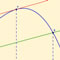Mean Value Theorem 4.2 An illustration of the Mean Value Theorem. Rolle's Theorem is included as a special case.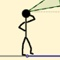Optimization - Art Gallery 4.7 A visualization of a classic optimization problem: Where is the best spot to stand to view a painting?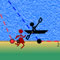Optimization - Rowing and Running 4.7 A visualization of a classic optimization problem.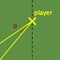Optimization - Soccer 4.7 Investigate the optimum position on the soccer field to score a goal.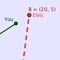Optimization - Elvis the Dog 4.7 Elvis the Calculus Wonderdog!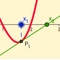Newton's Method 4.8 A dynamic illustration of Newton's Method for approximating roots of functions.Newton's Method Implementation 4.8 Implementation of Newton's Method. Don't bother using your calculator to crunch the numbers, instead use a computer - it is what they are great for. Just change the function and the initial value and compute away!

Here is a pure python version in a jupyter notebook format: (view) (download: .ipynb)

Parametric Curves and Polar Coordinates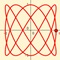Circle, Ellipses and Lissajous Parametric Curves Applet 10.1 Some of the most basic parametric curves. Here we see that circles, ellipses and Lissajous curves all arise from sine and cosine through parametrizations.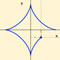Parametric Curves Applet 10.1 This applet is to help you visualize how a parametric curve is constructed from two individual functions: one for the x coordinate and one for the y-coordinate.

(currently this one doesn't work on all mobile devices - it needs java to run)

Here is another applet in which you can plot polar curves [java] [html5].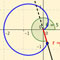Polar Curves Plotter 10.3 Just a polar curve grapher. Type in your polar equation and investigate the graph.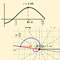Polar Curves and Cartesian Graphs 10.3 An applet showing the connection between the Cartesian graph of r=f(θ) and the graph in polar coordinates.

Integral Calculus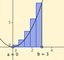Riemann Sums Applet 5.1 This applet is to help you visualize Riemann sums. You can change the function, move the sliders to change from left to right Riemann Sums, and increase the number of rectangles. Notice the more rectanges you use, the closer they approximate the actual area under the curve.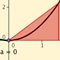Approximate Integration Applet 7.7 This applet is to help you visualize the techniques we have developed for approximating integrals: left-endpoint, right-endpoint, and midpoint methods, trapezoid rule, and Simpson's rule.Implementations of lefthand, righthand, and midpoint rules, trapezoid rule, and Simpson's rule. Don't bother using your calculator to crunch the numbers, instead use a computer - it is what they are great at. Just change the function, interval and n, and compute away!Infinite Series: Approximations to famous mathematical constants - by Nils BruinTools for visualizing differential equations: Vector Field Plotter - by Nils Bruin